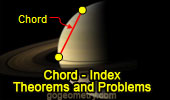# Online Plane Geometry: Chord of a Circle, Theorems and Problems

 Circle Chord, Theorems and Problems 10Proposed Problem 275. Right Triangle, Circumcircle, Sagitta, Inradius, Chords. Proposed Problem 262. Regular Hexagon inscribed in a circle, sum of distances, Chords. Proposed Problem 261. Regular Pentagon inscribed in a circle, sum of distances, Chords. Proposed Problem 257. Equilateral Triangle, Circumcircle, Chords, Point, Vertices, Side, Distances, Squares. Proposed Problem 256. Equilateral Triangle, Circumcircle, Point, Vertices, Distances, Chords. Proposed Problem 215. Quadrilateral, Angle Bisectors, and Cyclic Quadrilateral, Chords. Proposed Problem 208. Triangle, Excircles, Angles, 360 degrees, Chords. Proposed Problem 181. Circular Sector of 90 degrees, Chords, find an angle. Go to Page: Previous | 1 | 2 | 3 | 4 | 5 | 6 | 7 | 8 | 9 | 10 | Next

 Home | Geometry | Circle | Email | Post a comment | By Antonio Gutierrez